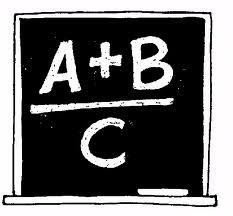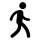# Equation

Find x:

x + 1/2 = 1/3

x =  -0.1667

### Step-by-step explanation:

x + 1/2 = 1/3

6x = -1

x = -1/6 ≈ -0.166667

Our simple equation calculator calculates it.Did you find an error or inaccuracy? Feel free to write us. Thank you!Tips to related online calculators
Need help to calculate sum, simplify or multiply fractions? Try our fraction calculator.
Do you have a linear equation or system of equations and looking for its solution? Or do you have a quadratic equation?

## Related math problems and questions:

• Find unknown 2Find unknown denominator: 2/3 -5/? = 1/4
• Simplify 7Simplify. 7-1/3÷ 3-2/3 of 2+ 4-1/2÷ 2-1/4+ 1/2 solution and by step by step
• Reciprocal equation 3Solve reciprocal equation: 1/2 + 2/3=1/x
• EquationSolve equation and check the result: 1.4x - 3/2 + x - 9,8 = x + 0,4/3 - 7 + 1,6/6
• Missing numberBlank +1/6 =3/2 find the missing number
• Fractions and mixed numerals(a) Convert the following mixed numbers to improper fractions. i. 3 5/8 ii. 7 7/6 (b) Convert the following improper fraction to a mixed number. i. 13/4 ii. 78/5 (c) Simplify these fractions to their lowest terms. i. 36/42 ii. 27/45 2. evaluate the follow
• RunningJoanne ran 8 1/2 Kyleigh 1 2/3 and John 6 3/4 miles in one day. How many miles did the girls run in 3 days?
• Equivalent fractions 2Write the equivalent multiplication expression. 2 1/6÷3/43 3/4 + 2 3/5 + 5 1/2 Show your solution.
• Fraction of a NumberIf 1/2 of 1/3 of 1/4 of 1/5 of a number is 2.5. what is the number?
• Evaluate mixed expressionsWhich of the following is equal to 4 and 2 over 3 divided by 3 and 1 over 2? A. 4 and 2 over 3 times 3 and 2 over 1 B. 14 over 3 times 2 over 7 C. 14 over 3 times 7 over 2 D. 42 over 3 times 2 over 31
• Find the 24Find the difference between 2/7 and 1/21
• LCD 2The least common denominator of 2/5, 1/2, and 3/4
• The length 6The length of 12 pipes is 10 1/2 meters. (1) find the length of one pipe (2) also find the length of 7 pipes
• Simplify 3Simplify mixed numerals expression: 8 1/4- 3 2/5 - (2 1/3 - 1/4) Show your solution.
• Simple equation 2Find X in this simple equation: X/9 = 96/108
• Walking womanReeta walks 5/7 km in 1 hour. How far does she go in 3 1/2 hours?